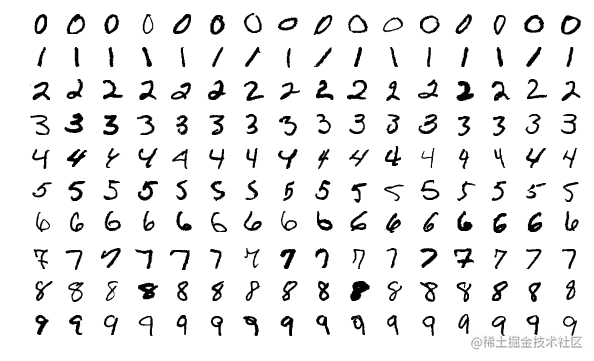# 学习 Pytorch 就像打游戏，想停也停不下来(1)• 分解任务问题
• 然后找到一个合适函数集，通常我们都是用具有一定结构网络来模拟复杂函数集
• 收集合适数据，数据需要能够正确反映我们任务
• 然后设定一个目标，对于神经网络就是损失函数，这样模型参数调整就用了方向
• 设定调整参数的策略，也就是优化器
• 设定，也就是我们任务更关心那些指标，这些指标可以反映任务能力，例如准确率、精准率和召回率

``````import torch
import torch.nn as nn

``````import torch.nn.functional as F

``````x = torch.randn(1, 1)
w = nn.Parameter(torch.randn(1, 1))

output = x * w
print(output)

``````tensor([[-0.1428]], grad_fn=<MulBackward0>)

``````output.backward()

``````tensor([[-0.2470]])``````from torch.utils.data import DataLoader

import torchvision.datasets as datasets
import torchvision.transforms as transforms

torch.utils.data 导入 DataLoader 用于加载数据集，随后我们会分享关于如何自定义数据集，torchvision 之前给大家介绍这个模块，是利用 pytorch 提供一些视觉方面预定义模型或者数据集，这些工具帮助我们方便地进行视觉方向研究。### 定义网络

``````class Net(nn.Module):
def __init__(self,input_size,num_classes):
super(Net,self).__init__()
self.hidden_dim = 30
self.fc1 = nn.Linear(input_size,self.hidden_dim)
self.fc2 = nn.Linear(self.hidden_dim,num_classes)

def forward(self,x):
x = F.relu(self.fc1(x))
x = self.fc2(x)
return x

``````model = Net(784,10)
x = torch.randn(64,784)
print(model(x).shape)`x = torch.randn(64,784)` 中这里 64 表示一个批次输入大小为 64 个样本，然后对于每一个样本会输出其属于 10 类别的概率分布。

``````torch.Size([64, 10])### 设定网络运行设备

``````device = torch.device('cuda' if torch.cuda.is_available() else 'cpu')

### 设置超参数

``````input_size = 784
num_classes = 10
learning_rate = 0.001
batch_size = 64
num_epochs = 1

### 准备数据集

``````train_dataset = datasets.MNIST(root='dataset/',train=True,transform=transforms.ToTensor(),download=True)

### 初始化模型

``````model = Net(input_size=input_size,num_classes=num_classes).to(device)

### 损失函数和优化器

``````criterion = nn.CrossEntropyLoss()

``````criterion = nn.CrossEntropyLoss()

### 训练模型``````for epoch in range(num_epochs):
data = data.to(device)
targets = targets.to(device)
print(data.shape)
break

``````torch.Size([64, 1, 28, 28])

64 表示每一个批次提供图像数量、1 表示图像的通道数和 28 和 28 分别代表图像宽和高度

``````for epoch in range(num_epochs):
data = data.to(device)
targets = targets.to(device)
data = data.reshape(data.shape,-1)

# 前向传播
pred = model(data)
loss = criterion(pred,targets)

# 反向传播
loss.backward()

# 更新参数
optimizer.step()

### 验证

``````def validation_acc(loader,model):
num_correct = 0
num_samples = 0
model.eval()

x = x.to(device)
y = y.to(device)

x = x.reshape(x.shape,-1)

y_hat = model(x)
_,pred = y_hat.max(1)

num_correct += torch.eq(pred,y).sum()
num_samples += pred.size(0)
print(f"acc {float(num_correct)/float(num_samples)}")
acc = float(num_correct)/float(num_samples)
model.train()
return acc

``````validation_acc(test_loader,model)

``````acc 0.9215